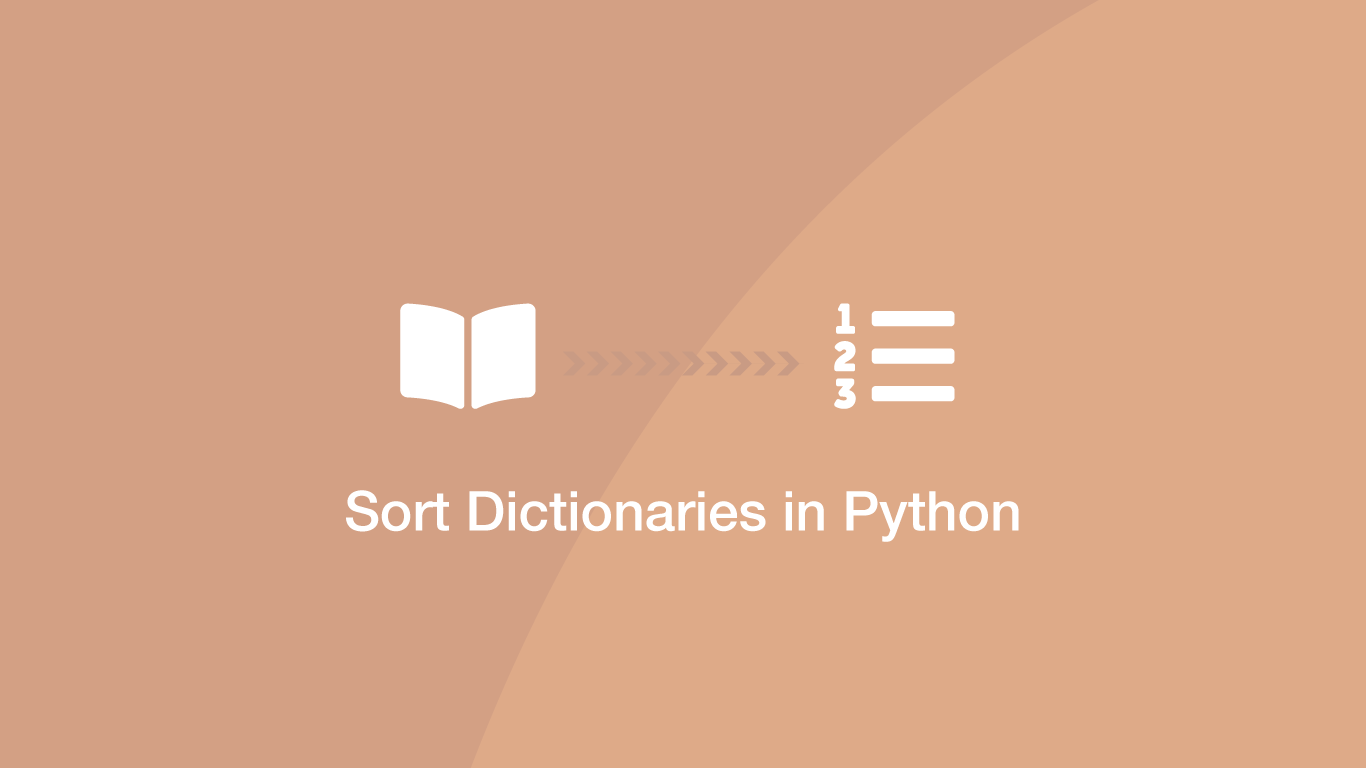# How to Sort Dictionaries by Value In PythonDictionaries in Python are used to store data in a key/value pattern. It is possible to sort dictionaries in a variety of different ways using the `sorted()` function, which is what we will be learning to do in this tutorial.

If you are unfamiliar with how dictionaries work, read how to use dictionaries in Python.

## The sorted() Syntax

The sorted() function has one required argument and two optional arguments. It will return a new `list` containing sorted data.

``````sorted(iterable, [key, [reverse]])
``````

• `iterable` - the iterable object containing the values that are to be sorted
• `key` - use a custom sort key (optional)
• `reverse` - if set to True the order of the new list will be reversed (optional)

## Sort a Dictionary by Key

Let's begin by sorting a dictionary by its keys. This is what `sorted()` will look for by default so no other arguments are needed.

``````dictionary = {'this': 4, 'sentence': 1, 'of': 1, 'cats': 3, 'dogs': 2}

s = sorted(dictionary)

print(s)
``````
``````['cats', 'dogs', 'of', 'sentence', 'this']
``````

## Sort a Dictionary by Values

To sort a dictionary by values, we will first have to convert the list to a list of tuples (the tuples being the key/values from the dictionary) using the `.items()` method. Then we will set the `key` using a lambda function which will get the second element from the tuple (the value).

``````dictionary = {'this': 4, 'sentence': 1, 'of': 1, 'cats': 3, 'dogs': 2}

s = sorted(dictionary.items(), key=lambda x: x)

print(s)
``````
``````[('sentence', 1), ('of', 1), ('dogs', 2), ('cats', 3), ('this', 4)]
``````

## Sort in Descending Order

To sort a list in descending order, pass the `reverse=True` as the third argument of the `sorted()` function.

``````dictionary = {'this': 4, 'sentence': 1, 'of': 1, 'cats': 3, 'dogs': 2}

s = sorted(dictionary.items(), key=lambda x:x, reverse=True)

print(s)
``````
``````[('this', 4), ('cats', 3), ('dogs', 2), ('sentence', 1), ('of', 1)]
``````

## Conclusion

You now know to sort dictionaries by its values in Python. Probably the only part that can be a little tricky to wrap one's head around is the use of the lambda function to get the value from the tuple and set it as the key. If you check out the article I linked to about anonymous functions hopefully it will make more sense.

#### Related Tutorials### Pretty Print a Dictionary in Python

September 16, 2021### HTTP Requests in Python (GET, POST, PUT, PATCH, DELETE)

October 06, 2020### How to Calculate Mean, Median, Mode and Range in Python

October 07, 2020### How to Parse JSON Data in Python (Read and Write)

September 18, 2020### How to Create and Use Lists (Arrays) in Python

September 01, 2020June 10, 2021